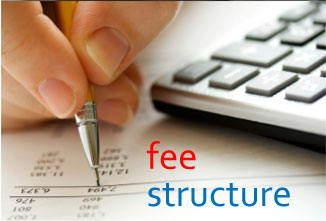FEE StructureSr. No.

Year

OPEN (Fees in Rs.)

SC

OBC

ST

VJNT/SBC

TFWS

OMS

1.

First Year ( tentative)

42,000=00
(22,995=00 if EBC)

00=00

22,995=00

3,991=00

3,991=00

3,991=00

42,000=00

2.

Direct Second Year(tentative)

35,000=00
(18,856=00 if EBC)

00=00

18,856=00

2,712=00

2,712=00

NA

35,000=00